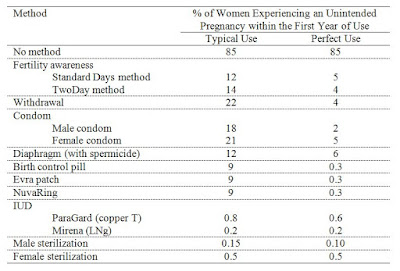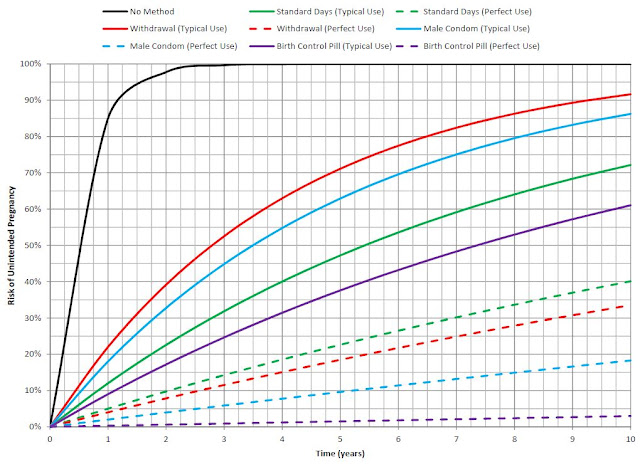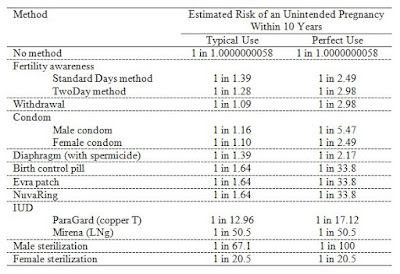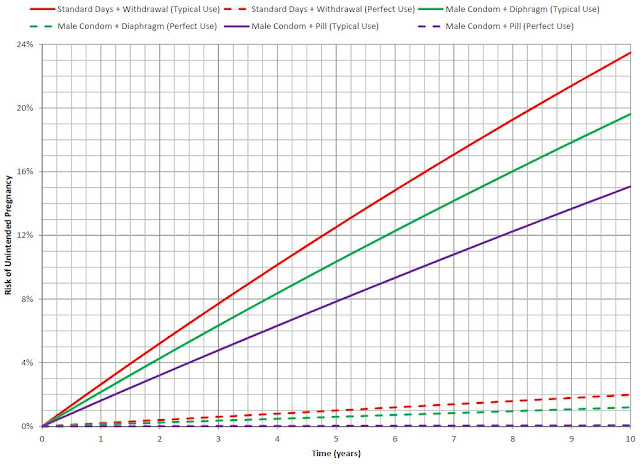## Monday 28 September 2015

### Contraceptive Efficacy and Combined Probability

When sex education sources quote numbers on the efficacy of different forms of contraception, they usually report a number like "98% effective". What they really mean is that, on average, 98 out of 100 women using that method of birth control for a whole year won't get pregnant. However, most people don't understand how a seemingly small annual risk of pregnancy might translate to a significant risk of pregnancy over a period of several years. In this post I show you how the probability of pregnancy grows over time and why it's commonly recommended that couples use two forms of contraception.

The annual risk of unintended pregnancy for several different contraceptive methods are given in the table below, taken from the 20th edition of Contraceptive Technology. The effectiveness typically reported by sex education sources is simply 100% minus the annual risk of pregnancy.I'm not sure how typical use differs from perfect use when it comes to male sterilization...

It should be noted that "typical use" is perhaps misleading because it is an average for the people reportedly using that contraceptive method. For most contraceptive methods, including the condom and the pill, the most common deviation from "perfect use" is conscious user non-compliance (i.e. knowingly not using the contraception). Not using the contraception shouldn't really count in the typical use, but I guess they just do the best they can with the data they get.

So, suppose you're interested in calculating the risk of pregnancy within a period several years. From the table above, we can get the probability of not conceiving during the first year of use, equal to 100% minus the number from the table. We'll call this number p. The probability of not conceiving during a period of n years of use can be taken as p raised to the nth power. The risk of pregnancy is then calculated as

This simply estimates the risk of getting pregnant at least once during an n-year period while using a certain contraceptive method. The graph below compares a few common contraceptive methods for n = 1 to 10 years.Comparing the risk of pregnancy using different contraceptive methods.

The graph shows that if you are not diligent in using the contraceptive method properly (i.e. you stray from "perfect use"), there's a pretty good chance of unintended pregnancy somewhere down the road. There's greater than 50% chance of unintended pregnancy within 8 years of "typical use" with the pill, within 6 years with the Standard Days method, 4 years with the male condom, and 3 years with the withdrawal method. Even with perfect use, if condoms are the only contraceptive method used, there's still about 18.3% chance of an unintended pregnancy in 10 years. That's 1 in 5.47. If you look at a 10-year period, comparing all the methods from the previous table, you'll find that if you use only a single method, only sterilization or IUDs reduce your risk of unintended pregnancy to less than 1 in 2 (i.e. less than 50%), assuming "typical use".We can estimate the risk of pregnancy when contraceptive methods are combined by combining probabilities as shown in the following equation:

When two events, A and B, are independent, the probability of both A and B occurring is equal to the product of the probability of A and the probability of B. Applied to pregnancy risk, it means the risk of getting pregnant while using both methods A and B is equal to the probability of A failing multiplied by the probability of B failing. This approach to combining probability assumes that the individual probabilities are independent of each other. Before combining probabilities you should think about whether they actually are reasonably independent. For example, birth control pills and male condoms are probably independent (or nearly independent) because they are unrelated methods and it is unlikely that one method will influence the efficacy of the other. Therefore, the probability of an unintended pregnancy in the first year of use is probably approximately 0.18*0.09 = 1.62% when both male condoms and birth control pills are used (assuming "typical use"). A counterexample is the combination of the Standard Days Method and the TwoDay Method, which are probably not independent because they are both methods of achieving "fertility awareness". It's unlikely that one method negatively influences the other, but combining the two probably has little benefit either. The combination of Standard Days Method with the TwoDay Method is probably only slightly better than either one of them their own. That said, I've calculated the risk of pregnancy with some combinations of contraceptive methods which probably satisfy the assumption of independence in order to demonstrate how using two methods simultaneously dramatically reduces your risk of unplanned pregnancy.Comparing the risk of pregnancy using different combinations of contraceptive methods.

As you can see, combining contraceptive methods reduces the risk of unintended pregnancy by a pretty significant margin. If using a single contraceptive method, assuming "typical use", only IUDs or sterilization were effective enough to reduce the risk of unintended pregnancy to less than 50% in 10 years. Combining 2 methods very effectively reduces the risk of unintended pregnancy without resorting to invasive or irreversible medical procedures.